Published

# Power Wheels

Over the span of the Spring 2023 semester, Team 8 utilized a children's power wheel to implement and test various sensors.

IntermediateWork in progress11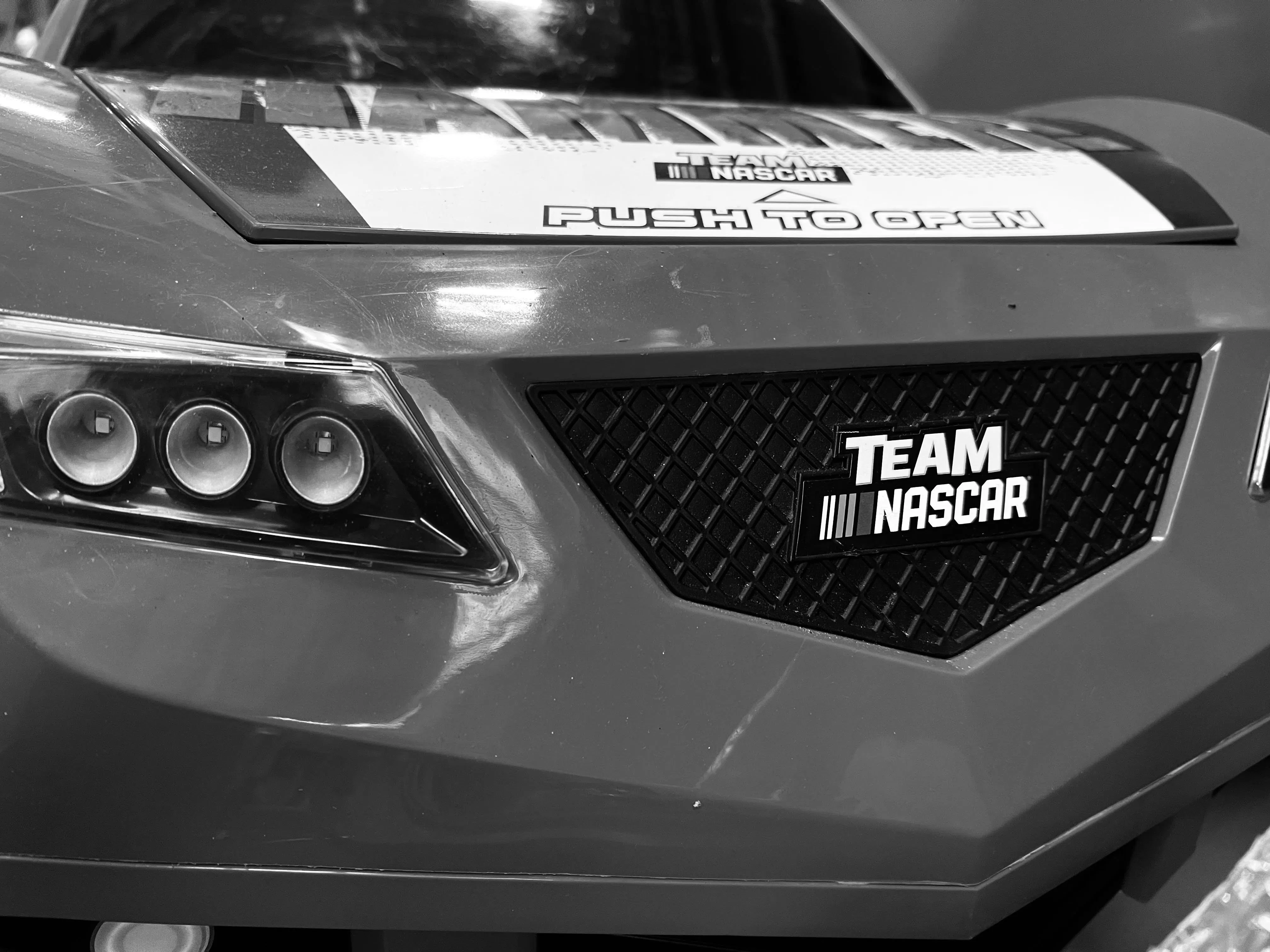## Things used in this project

### Hardware components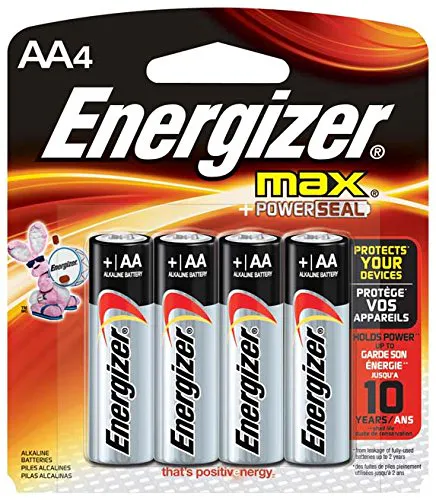AA Batteries
×1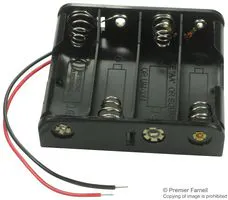Battery Holder, AA x 4
×1×1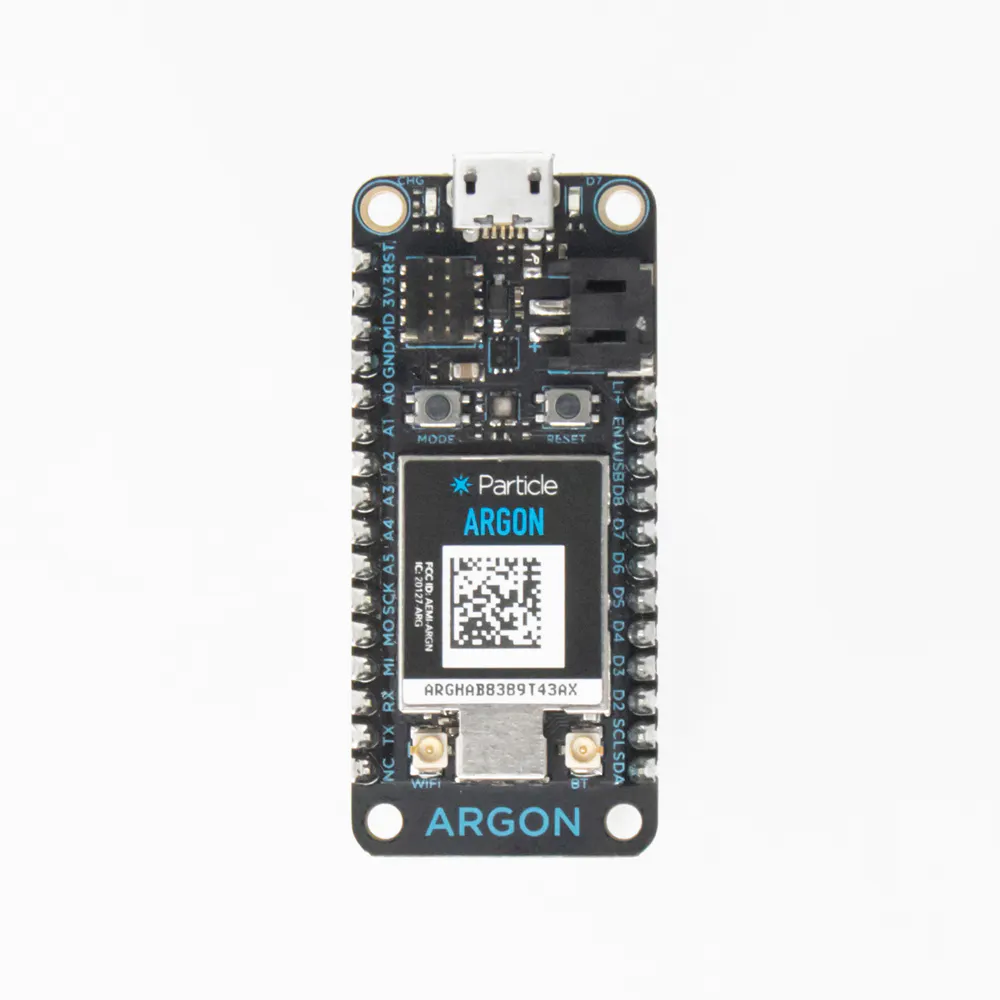Particle Argon
×1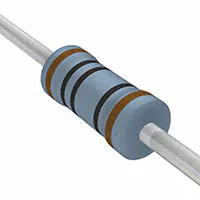Resistor 100 ohm
×4Resistor 10k ohm
×1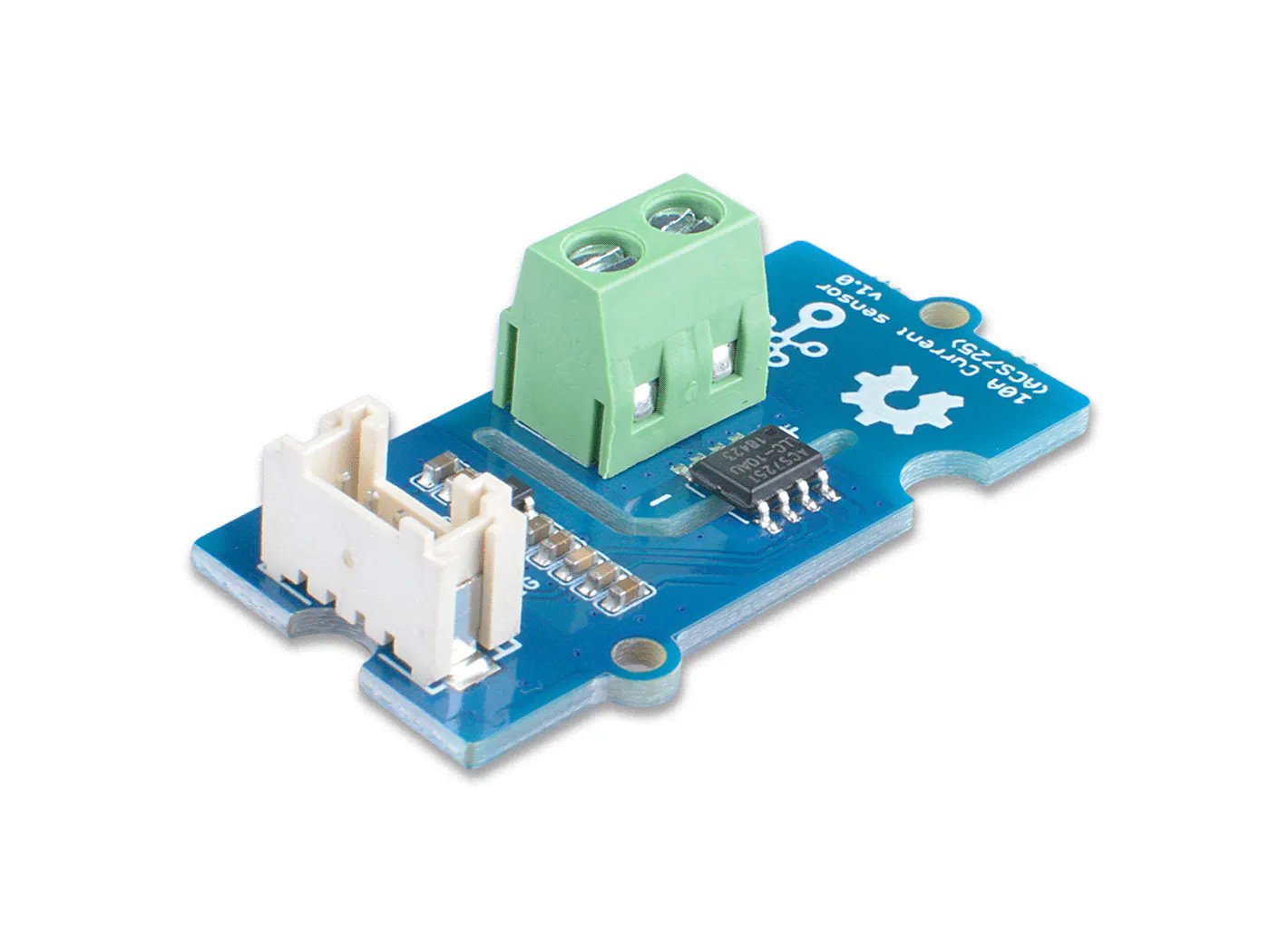Seeed Studio Grove - 10A DC Current Sensor (ACS725)
×1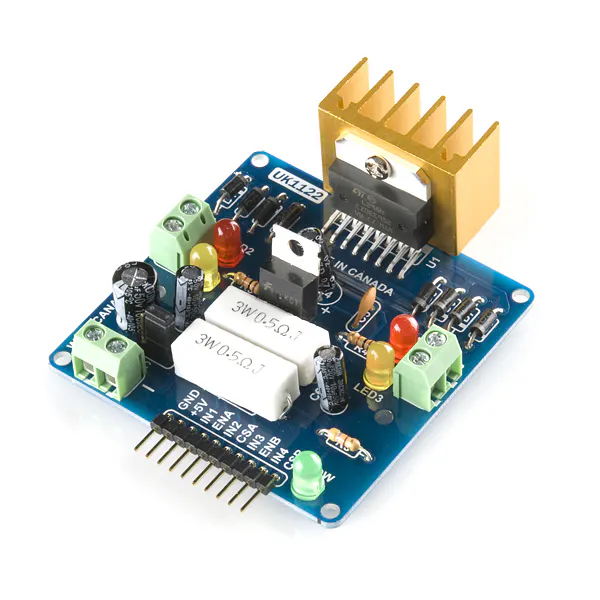SparkFun Dual H-Bridge motor drivers L298
×1
 Throttle Sensor
×1

### Software apps and online servicesParticle Build Web IDE

### Hand tools and fabrication machines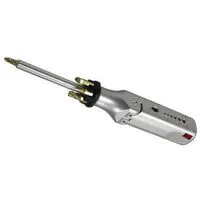Multitool, Screwdriver

## Schematics

### Power Wheel Schematic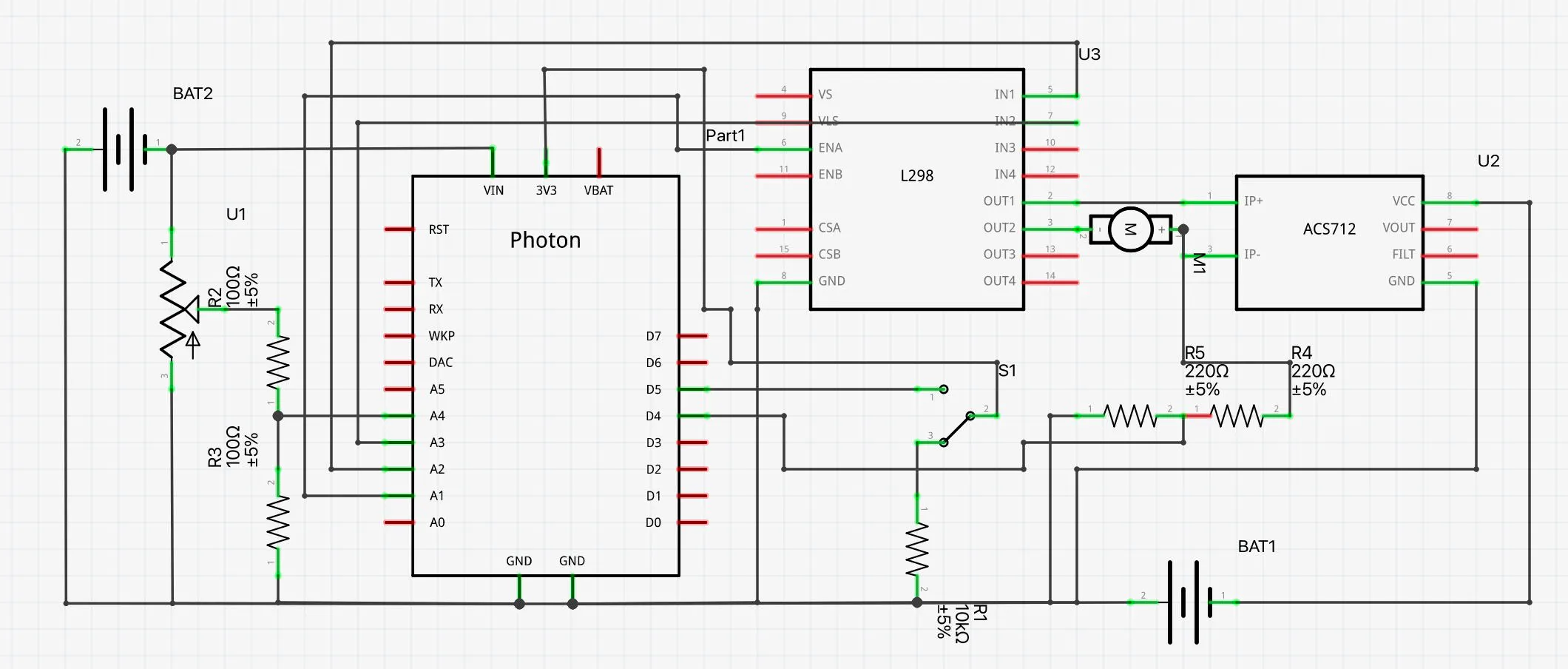## Code

### Slew Rate Traction Control

C/C++
```%Slew Rate Traction Control%#define enA A1
#define in1 A2
#define in2 A3

int rotDirection = 0;
int potValue = 0;
int smoothedpwm = 0;
int pressed = false;

//int button = D5;

void setup() {
pinMode(enA, OUTPUT);
pinMode(in1, OUTPUT);
pinMode(in2, OUTPUT);
pinMode(D5, INPUT_PULLDOWN);
// Set initial rotation direction
digitalWrite(in1, LOW);
digitalWrite(in2, HIGH);
Particle.variable("potValue", String(potValue));
Particle.variable("in1", String(in1));
Particle.variable("in2", String(in2));
Particle.variable("TPS", String(smoothedpwm));
Serial.begin(9600);
}

void loop() {
//Particle.publish("potValue", String(potValue), PRIVATE);
//Particle.publish("in1", String(in1), PRIVATE);
//Particle.publish("in2", String(in2), PRIVATE);
// Direction Control
if (pressed == true){
digitalWrite(in1, HIGH);
digitalWrite(in2, LOW);
delay(20);}
if (pressed == false){
digitalWrite(in1, LOW);
digitalWrite(in2, HIGH);
delay(20);}
// Speed Control
int pwmOutput = potValue; //map(potValue, 0, 255, 0, 255); // Map the potentiometer value from 0 to 255 potValue;
smoothedpwm += (pwmOutput - smoothedpwm)*0.1; // Adjust the constant scaling factor for different rates
Serial.println(smoothedpwm);
analogWrite(enA, smoothedpwm);
//Serial.println();
//Serial.println(pressed);
//Serial.println(in1);
//.println(in2);
Particle.publish("TPS", String(smoothedpwm), PRIVATE);
delay(50);
}
```

### Power Wheels Variable Throttle Sensor

C/C++
```#define enA A1
#define in1 A2
#define in2 A3

int rotDirection = 0;
int potValue = 0;
int smoothedpwm = 0;
int pressed = false;

//int button = D5;

void setup() {
pinMode(enA, OUTPUT);
pinMode(in1, OUTPUT);
pinMode(in2, OUTPUT);
pinMode(D5, INPUT_PULLDOWN);
// Set initial rotation direction
digitalWrite(in1, LOW);
digitalWrite(in2, HIGH);
Particle.variable("potValue", String(potValue));
Particle.variable("in1", String(in1));
Particle.variable("in2", String(in2));
Particle.variable("TPS", String(smoothedpwm));
Serial.begin(9600);
}

void loop() {
//Particle.publish("potValue", String(potValue), PRIVATE);
//Particle.publish("in1", String(in1), PRIVATE);
//Particle.publish("in2", String(in2), PRIVATE);
// Direction Control
if (pressed == true){
digitalWrite(in1, HIGH);
digitalWrite(in2, LOW);
delay(20);}
if (pressed == false){
digitalWrite(in1, LOW);
digitalWrite(in2, HIGH);
delay(20);}
// Speed Control
int pwmOutput = potValue; //map(potValue, 0, 1023, 0 , 255); // Map the potentiometer value from 0 to 255
smoothedpwm += (pwmOutput - smoothedpwm)*0.1;
//Serial.println(smoothedpwm);
analogWrite(enA, smoothedpwm);
Serial.println();
Serial.println(pressed);
Serial.println(in1);
Serial.println(in2);
Particle.publish("TPS", String(smoothedpwm), PRIVATE);
delay(5000);
}
```

### Current Sensor

C/C++
```float currentCurrent = 0;
float voltageCheck = 0;
void setup() {
Particle.variable("Current", String(currentCurrent));
Particle.variable("Voltage", String(voltageCheck));
}
void loop() {
if (currentCurrent>0) {
Particle.publish("currentCurrent", String(currentCurrent), PRIVATE);
}
if (voltageCheck>0) {
Particle.publish("voltageCheck", String(voltageCheck), PRIVATE);
}
delay(10000);
}
```

## Credits

### Cherokee Hall

1 project • 1 follower
Thanks to Gus De Piante, Wesley Goodwin, and Carson Andrijanoff.